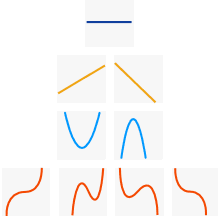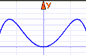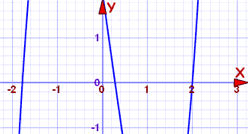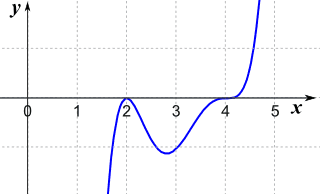# 解多项式多项式例子

## 求解

"求解" 的意思是求 "根"……

……"根"（或 "零点"）是函数等于零的点## 次数

……变量最大的指数。

###0 常数 71 线性 4x+3
2 二次 x2−3x+2
3 三次 2x3−5x2
4 四次 x4+3x−2 。。。
。。。 。。。

## 怎样去解

### 例子：2x+1

2x+1 是个线性多项式：y = 2x+1 的图是条直线

"根" 是 y 等于零的地方：2x+1 = 0

x = −1/2

（你也可以在图上看到答案）

### 例子： x3+2x2−x

x3+2x2−x = x(x2+2x−1)

### 例子：x3−8

x3−8 = x3−23

x3−8 = (x−2)(x2+2x+4)

x=2 是个根，因为：

(2−2)(22+2×2+4) = (0)(22+2×2+4)

### 三、画图。我们可以把多项式输入到函数画图器，然后扩大图象来看看在哪里与 x轴交叉。

## 因式

f(x) = (x−a)(x−b)(x−c)……，

（去看因式定理来了解更多。）

### 例子：f(x) = (x3+2x2)(x−3)

f(x) = (33+2·32)(3−3)

f(x) = (33+2·32)(0)

## 怎样检验

### 例子： 2x3−x2−7x+2f(2) = 2(2)3-(2)2−7(2)+2 = 16−4−14+2 = 0

f(−1.8) = 2(−1.8)3-(−1.8)2−7(−1.8)+2 = −11.664−3.24+12.6+2 = −0.304

### 例子（续）：2x3−x2−7x+2

f(2)=0 是个根……所以我们也找到一个因式：

(x−2)2x3−x2−7x+2 的因式

2x3−x2−7x+2 = (x−2)(2x2+3x−1)

…… 有 n 个根（零点）

3 个根。

## 可是，有些可能是复根1 1 1 实根
2 2 2 实根， 2 复根
3 3 3 实根， 1 实根和 2 复根
4 4 4 实根， 2 实根和 2 复根， 4 复根
etc   等等！

## 根的重数

### 例子：f(x) = (x−5)3(x+7)(x−1)2

f(x) = (x−5)(x−5)(x−5)(x+7)(x−1)(x−1)

(x−5) 用了 3 次，所以 "5" 是个"重"根，重数是 3，同样， (x+7) 出现了一次， (x−1) 出现两次，所以：

• +5 是个"重"根，重数是 3
• −7 是个"单"根，重数是 1
• +1 是个"重"根，重数是 2

• 若重数是偶数，曲线刚好在 "r" 接触 x轴（曲线在 x轴的一边）
• 若重数是奇数，曲线在 "r" 经过 x轴 （从 x轴的一边到另一边）

### 例子：f(x) = (x−2)2(x−4)3

(x−2)偶重数，所以曲线在 x=2 刚好接触 x轴

(x−4)奇重数，所以曲线在 x=4 经过 x轴## 总结

• 我们可以直接解一次（线性）多项式及二次多项式
• 三次或以上的多项式，画图可能会有帮助
• 这些也会有帮助：
• 知道根离开零最远有多远
• 知道总共有几个根（等于多项式的次数）
• 估计有几个复根、正根和负根
• 重数是一个根在分解里出现的次数。